# High School Physics : Understanding Elastic and Inelastic Collisions

## Example Questions

### Example Question #1 : Understanding Elastic And Inelastic Collisions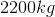car travelling at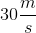collides with anothercar that is at rest. The two bumpers lock and the cars move forward together. What is their final velocity?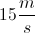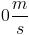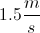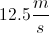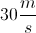Explanation:

This is an example of an inelastic collision, as the two cars stick together after colliding. We can assume momentum is conserved.

To make the equation easier, let's call the first car "1" and the second car "2."

Using conservation of momentum and the equation for momentum,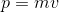, we can set up the following equation.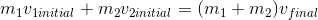Since the cars stick together, they will have the same final velocity. We know the second car starts at rest, and the velocity of the first car is given. Plug in these values and solve for the final velocity.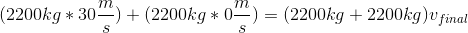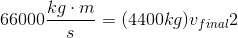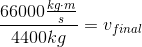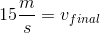### Example Question #2 : Understanding Elastic And Inelastic Collisions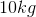ball moving at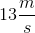strikes a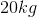ball at rest. After the collision theball is moving with a velocity of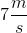. What is the velocity of the second ball?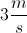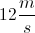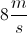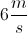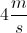Explanation:

This is an example of an elastic collision. We start with two masses and end with two masses with no loss of energy.

We can use the law of conservation of momentum to equate the initial and final terms.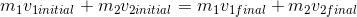Plug in the given values and solve for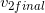.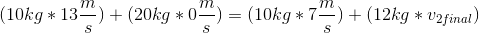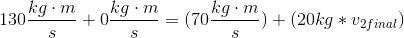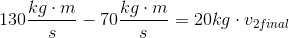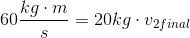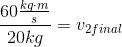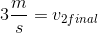### Example Question #1 : Understanding Elastic And Inelastic Collisions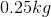ball strikes a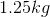ball at rest. After the collision theball is moving with a velocity of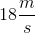and the second ball is moving with a velocity of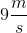. What is the initial velocity of the first ball?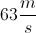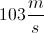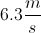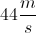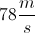Explanation:

This is an example of an elastic collision. We start with two masses and end with two masses with no loss of energy.

We can use the law of conservation of momentum to equate the initial and final terms.Plug in the given values and solve for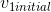.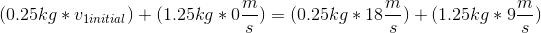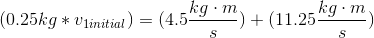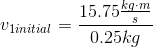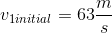### Example Question #301 : High School Physics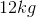ball moving at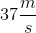strikes a second ball at rest. After the collision theball is moving with a velocity of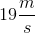and the second ball is moving with a velocity of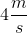. What is the mass of the second ball?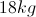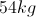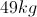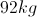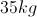Explanation:

This is an example of an elastic collision. We start with two masses and end with two masses with no loss of energy.

We can use the law of conservation of momentum to equate the initial and final terms.Plug in the given values and solve for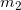.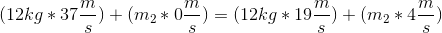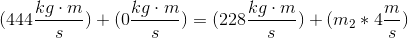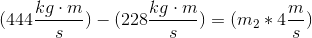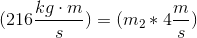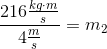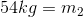### Example Question #1 : Understanding Elastic And Inelastic Collisions

Which of these would NOT be an example of an inelastic collision?

Two cars crash into each other, and stop with a loud bang

A man is clapping his hands such that they move with equal, but opposite velocities

Neutrons fuse with hydrogen atoms in a nuclear reactor core such that kinetic energy is conserved

Two hydrogen atoms fuse together to form a helium atom and gamma radiation

A match scrapes a matchbook and bursts into flame

Neutrons fuse with hydrogen atoms in a nuclear reactor core such that kinetic energy is conserved

Explanation:

The difference between an elastic and an inelastic collision is the loss or conservation of kinetic energy. In an inelastic collision kinetic energy is not conserved, and will change forms into sound, heat, radiation, or some other form. In an elastic collision kinetic energy is conserved and does not change forms.

Remember, total energy and total momentum are conserved regardless of the type of collision; however, while energy cannot be created nor destroyed, it can change forms.

In the answer options, only one choice preserves the total kinetic energy. The resulting bang from the car crash, the flame from the match, the sound of hands clapping, and the gamma radiation during hydrogen fusion are all examples of the conversion of kinetic energy to other forms, making each of these an inelastic collision. Only the neutron fusion described maintains the conservation of kinetic energy, making this an elastic collision.

### Example Question #1 : Momentum

Hockey puck A (2kg) travels with a velocity of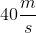to the right when it collides with hockey puck B (1.6kg), which was originally at rest. After the collision, puck A is stationary. Assume no external forces are in play and that the momentum of the pucks are conserved. What is the final velocity of puck B after the collision?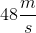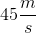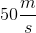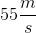Explanation:

Elastic collisions occur when two objects collide and kinetic energy isn't lost. The objects rebound from each other and kinetic energy and momentum are conserved. Inelastic collisions are said to occur when the two objects remain together after the collision so we are dealing with an elastic collision.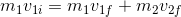Above, the subscripts 1 and 2 denote puck A and B respectively, and the initial momentum of puck B is zero, so that term is not included in the equation above.

Plug in initial and final velocities and mass: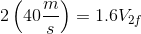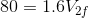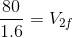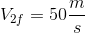### All High School Physics Resources